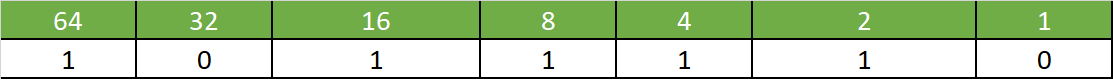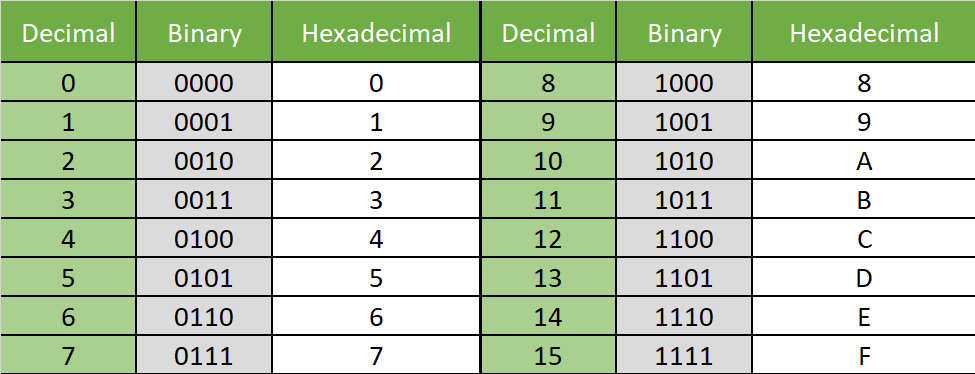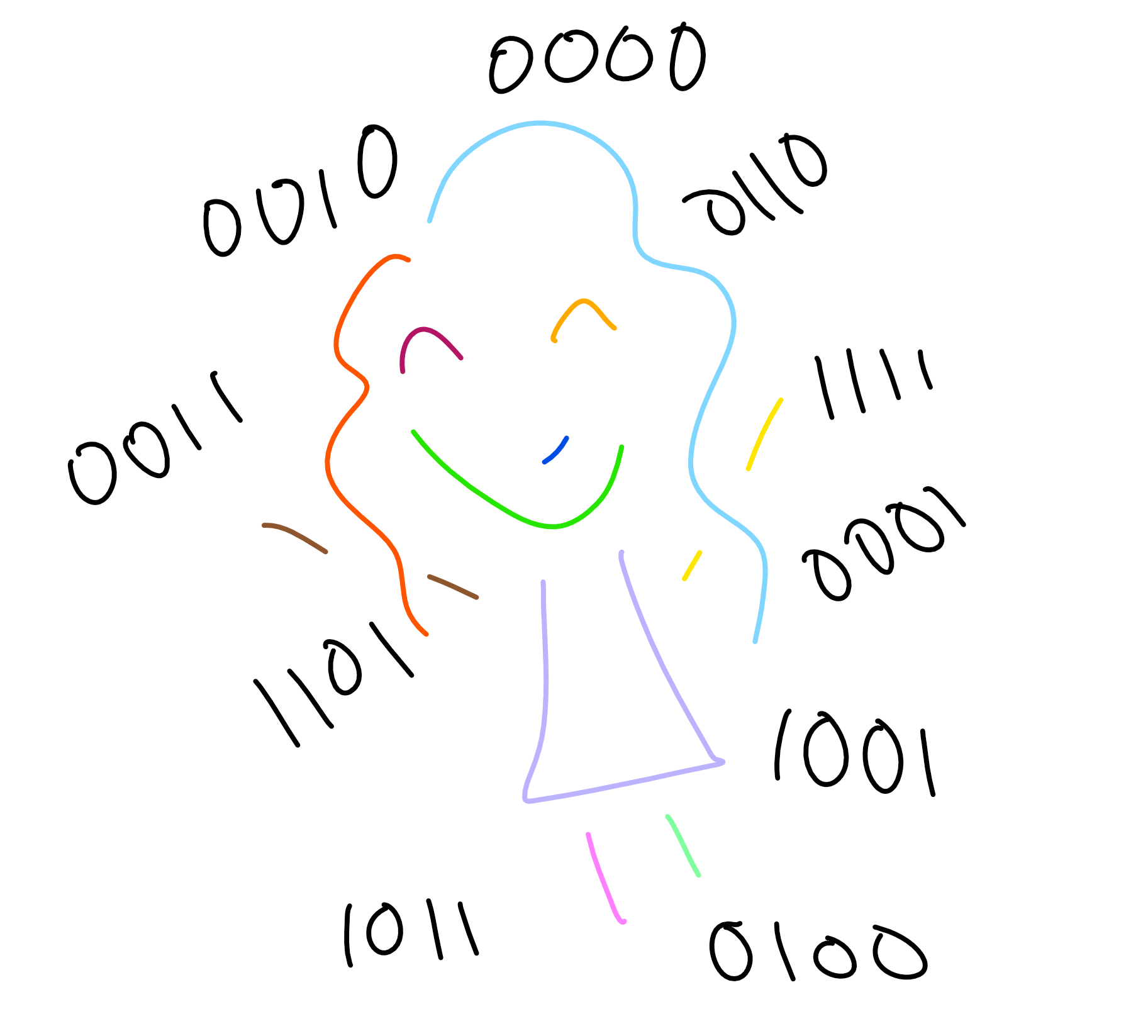So, I finally reached a point in my software development education where I could no longer avoid it. I needed to actually learn what binary was… Now a lot of you are probably sat here wondering how on earth I managed to get to this point without knowing, and to be honest, I have no excuse for you. My vague embarrassment has been somewhat compounded this morning by the fact that everything I needed to learn was included in GCSE bitesize, which made me feel older and more inadequate than I would have liked on a Tuesday morning. However, I can proudly say that I now have a grasp on the basics of how these things all fit together, and I'm also kicking myself for not having done this before because of how nice and satisfying it all is. I've very much enjoyed my morning's exploration.

So, (and I'm starting from the real basics here) generally we work in base 10. This means we have 10 characters – 0,1,2,3,4,5,6,7,8,9. Binary and hexadecimal systems work in base 2 and 16 respectively, so this means…

## Binary

Binary works in base 2, meaning there are only two characters: 0 and 1. With only one digit, there are then only two combinations, 0 or 1. With two digits you can have 00, 01, 10 or 11. This is 2² = 4 combinations. The same pattern holds in the decimal system – with only one digit there are 10 different combinations (0-9), then with two digits there are 10² = 100 combinations (0-99). When we reach 9 in the decimal system, we have to add an extra digit, and again binary follows the same pattern. In the decimal system each digit represents an extra power of 10. If you have the number 3425 you have 3 thousands (10³), 4 hundreds (10²), 2 tens (10¹) and 5 ones (10⁰). It works the same in binary, but using powers of 2. The first few integers, translated into binary are therefore:

Zero is fairly self-explanatory, but as we go up the pattern becomes clear. Take the integer 3 for example, it has one digit in the binary column corresponding to the value of 2, and one digit in the one column. Then, when we transition to 4, we have exhausted the maximum out of the first two digits and are forced to move into another digit (like when we reach 99 in the decimal system).So, to convert from binary to decimal you just need to remember that each digit corresponds to a power of 2. E.g. 1100111 = 1×64 + 1×32 + 0×16 + 0×8 + 1×4 + 1×2 + 1×1 = 103.

To do the conversion the other direction, there are two methods:

1. Take off the largest power of two that you can

e.g.

The first seven powers of 2 are: 1,2,4,8,16,32,64

For 94:

94 – 64 = 30 30 – 16 = 14 14 – 8 = 6 6 – 4 = 2 2 – 2 = 01. Divide by 2 and use the remainder

Again, for 94:

94 / 2 = 47 r 0         0 47/2 = 23 r 1           1 23/2 = 11 r 1           1 11/2 = 5 r 1            1 5/2 = 2 r 1             1 2/2 = 1 r 0             0 1/2 = 0 r 1             1

And then reverse the order:

94 = 1011110(as before)

So, in a computer the number of digits available is called a bit depth. If you have one bit of memory you only have one digit, so just two combinations. Often memory is measured in bytes, which corresponds to 8 bits of memory. This means that with one byte of memory you have 256 (2⁸) different combinations. The minimum integer which can be represented using 8 bits is zero (00000000) and the maximum is 255 (11111111).

Converting between binary and hexadecimal is extremely useful when working with computer memory. Sometimes in computing it is easier to see what is truly happening if you look at the binary numbers. However, working with binary is extremely cumbersome. Hexadecimal can therefore be used to see the binary structure without each number being tens of digits long. In hexadecimal you are working in base 16 and the conversions are as follows:Here we can see that every 4 bit binary number can be represented by a single hex digit. Therefore 8 bits (one byte) of computer memory can be represented by just two hex digits. E.g. 11010011 = 1101 0011 = D3.

Converting to binary and then to decimal is also the easiest way to convert hexadecimal into decimal. E.g. 4A = 0100 1010 = 0×128 + 1×64 + 0×32 + 0×16 + 1×8 + 0×2 + 1×2 + 0×1 = 74.Or the alternative is to use the base 16 columns: 4A = 4×16¹ + 10×16⁰ = 64+10 = 74. (Which of these I chose to use would I think depend on whether or not I had access to a calculator). The calculator app on windows actually has a really useful "programmer" mode which lets you work in decimal, binary, hex (and octal) and allows you to see all four representations of a number. Also (as I was confused by it when I first saw it) it is worth mentioning that 0x is the notation used in C-family programming languages to tell the compiler that a number is hexadecimal. I.e. 0x4A = 4A in hexadecimal = 74 in decimal.

Now, the final thing I wanted to mention (because I got very excited when I found out) was that the colour codes are all written in hexadecimal. Anyone that has done any web design will be aware that each colour can be represented by a 6-digit code: white is #FFFFFF, black is #000000, and everything else is somewhere in between (see where I'm going with this?). So, the first two digits represent how much red is present in the colour, the second two are green and the third pair are blue. Each pair represents a two-digit hexadecimal number, so FF (equivalent to 11111111 in binary) is the maximum for each colour and 00 is the minimum. Therefore, a colour that is pure red would be #FF0000.  As each pair is equivalent to one byte of memory, this means that for each colour there are 256 possible values. So, when combining our three colours there are 256×256×256 = over 16,000,000 possible colours!### Carmel Eve

#### Software Engineer I